﻿ Residence Time Distribution as a Tool for Hydrodynamic Study of Electrocoagulation Reactors Design and Scaling upPublications are Open
Access in this journal
Article Versions
Export Article
• Normal Style
• MLA Style
• APA Style
• Chicago Style
Original Article
Open Access Peer-reviewed

Residence Time Distribution as a Tool for Hydrodynamic Study of Electrocoagulation Reactors Design and Scaling up

Maryam Khadim Mbacké, Cheikhou Kane , Amintata Paye, Mouhamed Ndoye, Codou G. M. Diop
Chemical Engineering and Science. 2021, 6(1), 1-10. DOI: 10.12691/ces-6-1-1
Received August 28, 2021; Revised October 02, 2021; Accepted October 09, 2021

Abstract

An electrocoagulation reactor operating continuously is designed for the treatment of industrial effluents in Senegal. One of the main challenges for electrocoagulation reactors design is each reactor is specific for its domain. In this paper, a methodology using residence time distribution curve (RTD) is presented and can be used for any other examples. The optimization of the operating parameters such as the flow rate, the residence time, the conductivity and so on for the treatment of these effluents requires an understanding of the reactor hydrodynamics. Thus, this hydrodynamic study based on the residence time distribution curve (RTD) showed the presence of dead zone in the reactor with a theoretical residence time of 607.76 s for an average residence time obtained from the residence time distribution curve of 554.85 s. A comparison of the curve obtained experimentally with the curves for an ideal reactor of the piston type or of a perfectly stirred type, has shown that the electrocoagulation reactor behavior is like a cascade of two perfectly stirred reactors with a difference between them of 0.15%. The numerical simulation with COMSOL allowed to illustrate the velocity fields and to highlight the preferential zones during the flow in the reactor and thus to confirm the results obtained with the hydrodynamics with a relatively low absolute error of 4.6% obtained with the least squares method.

1. Introduction

In addition to technological and environmental aspects, cost-effective methods are required to treat a wide range of wastewater pollutants in a diverse range of conditions. Electrocoagulation (EC) is an emerging technology that combines the functions and advantages of conventional coagulation, flotation, and electrochemistry in water and wastewater treatment 1. As compared with traditional treatment methods, electrocoagulation provides a relatively compact and robust treatment alternative in which sacrificial metal anodes initiate electrochemical reactions that provide active metal cations for coagulation and flocculation. The inherent advantage of electrocoagulation is that no coagulants have to be added to the wastewater and hence the salinity of the water does not increase after treatment 2.

The technology is most commonly used in the oil and gas, construction, and mining industries to treat emulsified oil, total petroleum hydrocarbons, suspended solids, heavy metals, and other difficult-to-remove contaminants.

Electrocoagulation is performed by applying an electric current across metal plates that are submerged in water. Heavy metals, organics, and inorganics are primarily held in water by electrical charges. By applying another electrical charge to the contaminated water, the charges that hold the particles together are destabilized and separate from the clean water.

Electrocoagulation can be used as a pretreatment for processes such as clarification, reverse osmosis, and ultrafiltration, or as a polish treatment at the end of traditional treatment processes.

The technology, typically, eliminates the need of chemicals or biological additives or demulsifies. Without chemicals, there is also no need for chemical mixing tanks.

Previous studies have made it possible to assess the impact of the operating parameters on the efficiency of the electrocoagulation process 3. The study of the performance of an electrocoagulation reactor essentially consists of determining the degree of progress of the desired abatement reaction. The degree of progress of the reaction carried out by a reactor is the result of the combination of hydrodynamics and reaction kinetics.The residence time in the reactor, as well as the flow conditions have a significant influence on the purification performance. The understanding of the impact of these parameters requires an understanding of the hydrodynamic behavior of the reactor. In this study, the hydrodynamic behavior of an electrocoagulation reactor was tested with the residence time distribution method. The results obtained were compared to the ideal reactor models to understand the flows in the reactor. Comsol Multiphysics modeling software was used to simulate the reactor and confirm the experimental results.

The paper is organized as follows: in Section 1 we present the overall context of the study as well as the benefits of the electrocoagulation process. Section 2 presents the residence time distribution method and the principle of simulation with Comsol. Section 3 outlines our experimental setup and measurement procedures. Section 4 presents experimentally obtained results of the hydrodynamic measurements and simulation. Section 5 summarizes the hydrodynamic behavior of the reactor compared to the ideal reactor model.

Nomenclature

DTS residence time distribution

E(t) residence time distribution function (s-1)

E(t) dt fraction of the output flow which remained in the reactor for a time between t and t + dt.

F(t) cumulative distribution of residence times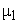first order moment of the residence time distribution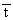mean residence time (s)

τ theoretical residence time (s)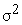variance or centered moment of order 2

C(t) variation of the concentration as a function of time at the outlet of the reactor (mg / L)

D dispersion coefficient (m2/s)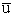mean flow velocity (m/s) (in the case of flow in a porous medium, this is the mean interstitial velocity)

φ (C) rate of production or consumption of the reagent (kg/m3.s)

Dz and Dr axial and radial dispersion coefficients (m2/s)Péclet number

L height of the section traveled between the inlet and the outlet (m)

n number of reactors

Γ(n) approximation of the Stirling formula of the gamma function

2. Theoretical Background

2.1. Résidence Time Distribution (RTD) Method

Residence time is an important parameter for performance evaluation used for characterizing, modeling, and optimizing a chemical reaction system. Continuous reactors, such as horizontal auger tubes, typically which have imperfect flow characteristics where undesired back-mixing and flow stratification lead to variations in residence time. This variation is characterized by the residence time distribution (RTD) curve, which describes the probability that a particle or chemical species will spend a specific time at desired reaction conditions. The variance about the mean time of this distribution is one indicator of how precisely the reactor is able to target a specific residence time 4.

The theory of residence time distributions generally begins with three assumptions: the reactor is at steady-state, transports at the inlet and the outlet takes place only by advection, and the fluid is incompressible.

The incompressibility assumption is not required always, but compressible flows are more difficult to work with. A further level of complexity is required for multi-phase reactors, where a separate RTD will describe the flow of each phase, for example bubbling air through a liquid. The distribution of residence times is represented by an external residence time distribution or an exit age distribution, E(t). The function E(t) has the units of time−1 and is defined such that:(1)
• Figure 1. An RTD curve for a reasonably well-mixed reactor

The fluid proportion that spends a given duration, t inside the reactor is given by the value of E(t)dt. The fraction of the fluid that leaves the reactor with an age time less that t1 is: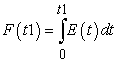(2)

where F(t) is called “cumulative distribution”. Thus, the fraction of the fluid that leaves the reactor with an age greater that t1 is: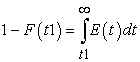(3)

The average residence time is given by the first moment of the age distribution:(4)

If there are no dead, or stagnant zones within the reactor, thenwill be equal to τ, the residence time calculated from the total reactor volume and the volumetric flow rate of the fluid: τV/v. The higher order central moments can provide significant information about the behavior of the function E(t). For example, the second central moment indicates the variance (σ2), the degree of dispersion around the mean is as follows: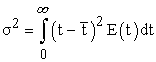(5)

Residence time distributions are measured by introducing a non-reactive tracer into the system at the inlet. The concentration of the tracer is changed according to a known function and the response is found by measuring the concentration of the tracer at the outlet. The selected tracer should not modify the physical characteristics of the fluid (equal density, equal viscosity) and the introduction of the tracer should not modify the hydrodynamic conditions. A suitable tracer material for RTD-experiments should exhibit similar physical properties, i.e. viscosity, diffusion coefficient, density, to the flow medium and in particular the materials used should behave inertly, not adhere or adsorb on to the reactor walls and be easily detectable. The experimental determination of the residence time distribution requires a well-defined introduction of a tracer species into the reactor inlet and the detection of its transient concentration leaving the reactor outlet. In general, the change in tracer concentration will either be a pulse or a step. Other functions are possible, but they require more calculations to deconvolute the RTD curve, E(t). In this study the pulse was used 5.

This method required the introduction of a very small volume of concentrated tracer at the inlet of the reactor, such that it approaches the dirac delta function. Although an infinitely short injection cannot be produced, it can be made much smaller than the mean residence time of the vessel. If a mass of tracer, M, is introduced into a vessel of volume V and an expected residence time of τ, the resulting curve of C(t) can be transformed into a dimensionless residence time distribution curve by the following relation: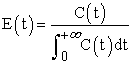(6)

The residence time distribution of a reactor can be used to compare its behavior to those of two ideal reactor models: the PFR and the CSTR (or mixed-flow reactor). This characteristic is important in order to calculate the performance of a reaction with known kinetics.

In an ideal PFR, there is no mixing and the fluid elements leave in the same order they arrived. One of the most frequently used models is a piston flow, on which is superimposed a dispersion mechanism. This model is sometimes called dispersive piston 6.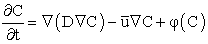(8)

With:

D: dispersion coefficient [m2s-1],: average velocity of the flow [m.s-1] (in the case of a flow in a porous medium, it is the average interstitial velocity),

φ (C): rate of production or consumption of the reagent.

In the case of incompressible flow in a cylindrical reactor, the axial symmetry makes it possible to define a longitudinal axis of ordinates z and a distance with respect to this axis r. In some cases, particularly when the radius of the reactor is small in front of the length, the effect of the radial dispersion can be neglect.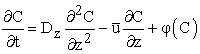(9)

In dimensionless coordinates and considering that the tracer does not react therefore φ (C) = 0, the equation 9 becomes: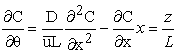(10)

This equation shows a dimensionless number called Peclet number: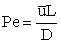(11)

The value of the number of Peclet gives indications on the type of flow:

1/Pe → 0 dispersion is negligible and the flow is piston type;

1/Pe → ∞ dispersion is important hence a mixed flow

If Pe <100 the dispersion is strong and the solution of the equation depends on the flow conditions at the entry and exit points of the tracer. When the entry point is a section throat, the tracer can not disperse upstream of the inlet section. On the other hand, if the input section of the tracer is identical to the section of the reactor, the tracer can disperse upstream. Similarly, if the exit section is strangled, the tracer can not return to the reactor by dispersion once it has passed the exit section. This is not the case if the output signal consists of a tracer sample in any section of the reactor.

In this study, the input and the output are opened to diffusion, which makes it possible to write the boundary conditions. Considering that the tracer is injected at z = 0 and its output concentration measured at z = L: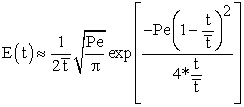(12)

The number of Peclet is obtained from the variance: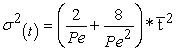(13)

The variance of an ideal plug-flow reactor is zero. The RTD of a real reactor deviate from that of an ideal reactor, depending on the hydrodynamics within the vessel. A non-zero variance indicates that there is some dispersion along the path of the fluid, which may be attributed to turbulence, a non-uniform velocity profile, or diffusion. If the mean of the E(t) curve arrives earlier than the expected time τ, it indicates that there is stagnant fluid within the vessel. If the RTD curve shows more than one main peak, it may indicate channeling, parallel paths to the exit, or strong internal circulation.

An ideal CSTR is based on the assumption that the flow at the inlet is completely and instantly mixed into the bulk of the reactor. The reactor and the outlet fluid have identical, homogeneous compositions at all times. An ideal CSTR has an exponential residence time distribution 7: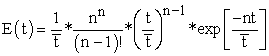(14)

To identify the model with an experimental curve, the only parameter to be determined is n, the average residence time being in principle given by the volume of liquid and the flow rate.

Whatever the method, it usually leads to a non-integer n.

When n is not integer, the physical meaning of the model is lost. However, it is possible to calculate the distribution using the gamma function.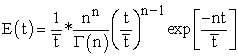(15)

To estimate the gamma function, we can use the approximation of the Stirling formula 8: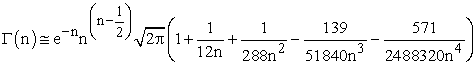(16)

When n is large, ie greater than 25, the SDRs calculated from the model of the n series reactors are superimposed on the SDRs calculated from the dispersive piston model, taking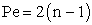(17)

The number of Peclet is obtained from the variance(18)
2.2. Principle of Simulation with COMSOL

The COMSOL software is one of the industrial CFD codes, allowing the prediction of fluids flow. It is a software that allows, in addition to dealing with multi physical problems. Indeed, the Partial Differential Equations (PDEs) are represented with equations in general forms allowing to personalize them and to couple them.

This software uses the finite element analysis method which is the most complete one to effectively solve the Navier-Stokes, convection-diffusion equations. The Navier-Stokes equations, which express physical laws of conservation of momentum, are deduced from the Newtonian laws of motion. They combine speed and pressure at each point of the flow 9.

Continuity equation: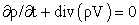(19)

Conservation of momentum equations: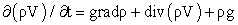(20)

It is possible to solve them using the model of the predefined rotational equations in COMSOL. This model will be used for 2D simulation.

3. Experimental Setup

To measure a distribution of the residence times, it is enough to "mark" the molecules to be able to detect them in the output stream for this the tracer used can be a radioactive body, a dye, or any substance with the same hydrodynamic properties as the fluid but detectable by a physical property such as electrical conductivity. A signal using the tracer is applied to the reactor inlet, so as not to disturb the flow regime, and the "response" to the output is examined.

The residence time distribution measurements were carried out by salt tracing with sodium chloride in a reactor with a volume occupied by the liquid of 6370 ml.

The sample taken to measure the concentrations at the outlet of the reactor must satisfy the conditions of representativeness of the concentration, in time and over the whole of the outlet section. The representativity with respect to the section is ensured either by a mixing device at the outlet of the reactor, or by adopting a small and small exit section with respect to the average section of the reactor. This option was chosen.

The choice of sampling frequency over time must be made accurately to prevent peaks or short circuits from being detected. To do this, preliminary tests with a maximum number of doses were made to set the frequency on the one hand and concentrate the catch in the vicinity of possible concentration peaks on the other hand.

The NaCl content at the outlet of the reactor is measured by a conductivity meter.

The reactor unit used at the pilot scale consists of four distinct zones. This is a first zone corresponding to the distribution chamber from which the effluent is distributed homogeneously in the cell. The second zone represents the electrocoagulation cell where the electrodes are housed. The third zone is a conical physical separation zone where the sludge is recovered allowing the flocs to be separated from the treated effluent. The fourth zone is used to recover the flocs that leave the cell under the effect of flotation.

• Figure 2. Experimental apparatus

A pump is used for the fluid flow in the reactor.

For simulation under COMSOL, a two-dimensional geometry is used with baffles to avoid preferential paths and to have a good time of stays. The simulation reactor is represented by the following Figure 3.

• Figure 3. 2D reactor geometry

4. Results and Discussions

Beforehand, the feed pump is calibrated to determine the actual flow rate (y) based on the flow rate displayed (x). The equation of the line reflecting the evolution of the conductivity(y) as a function of the concentration of sodium chloride (x) is also determined.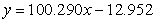(21)(22)

The tracing operation was carried out on the electrocoagulation reactor. The tracing being carried out with an injection of 1 g of NaCl in 5 ml of water with a flow rate of 10.48ml / s for a period of 5s, 98.48% of the mass of the tracer was restored.

• Figure 4. Residence time distribution in the reactor

The experimental results make it possible to determine the mean residence time calculated from the moment of order 1 (equation 4) and the variance is calculated with equation 5.

The theoretical residence time is given by the ratio of the volume to the flow rate, the reactor volume being 6370 ml and the flow rate 10.48 ml / s.

The average residence time obtained is less than the passage time<τ.

This result indicates a flow defect characterized by a short-circuit phenomenon. There is a preferential path in the reactor, which means that a fraction of the flow goes well before the rest. This corresponds to a large concentration peak in output just after the stimulation. The flow rate of fluid that then actually flows through the entire reactor is lower than that at the inlet.

Once the RTD curve has been obtained, its observation makes it possible to make some hypotheses. A peak or a large fluctuation of concentration in the first moments shows the presence of a short-circuit, an average residence time much lower than the passage time, the presence of a dead zone and a significant drag of the curve, the presence of stagnant zones.

The next step is to find a transfer function that is closest to the RTD curve, but not too complex to use in the future. This transfer function, coupled with the kinetic law of the reaction, makes it possible to evaluate the influence of the type of flow on the progress of the reaction at the outlet of the reactor.

The dispersive piston model (Equations 12 and 13) gave the following results with the variance and residence time obtained from the RTD curves.

• Figure 5. Residence times distribution of the dispersive piston model

Figure 5 shows a phase shift in time of the two distributions as well as a loss of amplitude of the experimental curve. This can be due to a recirculation zone in the reactor.

The cascade reactor model was tested with determination of reactor number n. The number of reactors is obtained from the variance.

Figure 6 shows the experimentally obtained RTD confrontation and the model of 2 cascaded reactors.

The results of the modelizations of the flow in a series of perfectly agitated reactors make it possible to draw different information on the hydrodynamic behavior of the reactor.

The comparison of the experimental and model RTD curves shows the reproduction of the shape of the curve with a loss of amplitude of the curve of the cascade model. This loss is probably due to the recirculation phenomenon and the presence of dead zones.

The values of the measured concentrations are quite close to the calculated values, the difference calculated by the least squares method is 0.15% for all the points. The final tail of the two curves induces the existence of dead zones.

The experimental results and the comparison with the dispersive piston reactor and cascade reactor models show that the system corresponds to a cascade of two perfectly stirred reactors. Indeed, the distribution chamber and the reaction chamber is like a series of reactors.

Simulation of flow with Comsol showed velocity profiles with preferential paths.

• Figure 6. Residence times distribution of the cascade model

Figure 7 confirms the presence of the vortices generated by the recirculation zones after widening of the passage section at the entrance and therefore the existence of negative speeds. This speed field confirms that the flow is far from evolving into piston flow.

In order to identify the zones of reciculation, the velocity profiles are represented at the inlet, at the middle and at the outlet of the reactor.

The profiles obtained do not indicate a negative value in the middle and at the exit. However, at the inlet of the reactor there are negative values of the speed, which shows that there is a recirculation zone.

The velocity which is practically nil at the bottom of the reactor reaches a maximum value in the middle of the reactor then increases to approximately 3.10-4 m / s when tending towards the reactor outlet.

The velocity fields obtained are used for the simulation of passive transport in the reactor.

It consists of injecting a concentration step C and simulating the evolution of the concentration in the reactor as well as at the outlet. The results given by COMSOL represent the flow of concentration at the exit by the section. This approximation is justified by the small diameter of the outlet compared to the diameter of the reactor.

The conditions of the simulations are the injection conditions of a step. At t = 0 the concentration in all the reactor is zero and then injected at the reactor inlet a concentration c which is equal to 3.42mol / l. At the output level a zero scalar flux is fixed.

• Figure 8. Profiles at the inlet, the middle and the outlet of the reactor
• Figure 9. Profile speed on a longitudinal plane
• Figure 10. Simulation of the evolution of the concentration

Figure 10 shows the evolution of the concentration in the reactor 1s after the step injection. Several simulations were performed at t = 50s, t = 100s, t = 200s, t = 500s, t = 750s, t = 1000s, t = 1500s and t = 1800s. From these simulations the concentration at the outlet was calculated for each case, which made it possible to trace the evolution of the concentration as a function of time in the reactor.

The experimental curve of the evolution of the concentration as a function of time with the same time steps is given in the figure below.

• Figure 11. Concentration profile obtained under COMSOL

The confrontation of RTD curves allows the comparison of calculations with experimental measurements. The evolution of the calculated concentrations regains the pace of the measurements (Figure 12) and the calculated values are in good agreement with the experimental values. However, there is a fairly large difference between the amplitudes. This difference is caused by the existence of dead zones confirmed by the simulation of hydrodynamics.

• Figure 12. Comparison of experimental RTD curves and simulation under COMSOL

5. Conclusion

The study of the hydrodynamics of the electrocoagulation reactor, through the determination of the residence time distribution, is a valuable tool for various reasons. Its first contribution is to allow exploration and understanding of flow and measurements hydrodynamics can also diagnose the causes of the malfunction to remedy it.

Sodium chloride is used as an injection tracer with a short time (5s) to approach a Dirac and the RTD obtained is used to validate the simulation models.

First, the experimental curve of the RTD is compared with the dispersive piston model: a phase shift as well as a loss of amplitude between the two curves are noted.

Then, the RTD is used to approach the cascade model of n perfectly stirred reactor; for n = 2 the model reproduces the shape of the experimental curve with a loss of amplitude, probably due to the existence of recirculation zones and dead zones, and the difference calculated by is 0.15%. This induces that the reactor has the behavior of a cascade of 2 perfectly stirred reactors with short circuit and dead zones.

To validate these obtained results, the COMSOL code was used to simulate the hydrodynamics of the reactor using the turbulence model k-ε. This simulation confirmed the existence of a recirculation zone in the distribution chamber and also dead zones.

The simulation of the passive transport is carried out in turbulent regime and the concentrations at the exit were calculated then the curve of the evolution of the distribution of the residence times was drawn, this curve reproduces the pace of the experimental curve with a loss amplitude and an error of 4.6% for the entire value series.

RTD modeling has well represented the hydrodynamic behavior of the reactor, the continuation of this study could improve the performance of this reactor: namely reduce dead zones and review the geometry to avoid recirculation of the fluid.

Acknowledgements

The authors would like to thank the Ministry of National Education and Research of the Republic of Senegal for financially supporting this research under Impulse Fund for Scientific and Technical Research.

References

  P. K. Holt, G. W. Barton and C. A. Mitchell, “The Future for Electrocoagulation as a Localised Water Treatment Technology,” Chemosphere, Vol. 59, No. 3, 2005 355-367. In article View Article  PubMed  I.Kadabsli, I. Arslan Alaton, T. Olmez Hanci, O. Tunay, Electrocoagulation applications for industrial wastewaters: a critical review, J. of environmental technology reviews 1, 3, 2012. In article View Article  M.K. Mbacké, C. Kane, I. Diouf, C.G.M.Diop Removal of crystal violet by electrocoagulation: Optimization and reaction mechanisms involved, J. Soc. Ouest-Afr.Chim. 39; 64-76 (2015) In article  D. Sievers, E. Kuhn, M. Tucker, J. Stickel, and E. Wolfrum Residence Time Distribution Measurement and Analysis of Pilot-Scale Pretreatment Reactors for Biofuels To be presented at the 2013 AIChE Annual Meeting San Francisco, California November 3-8, 2013. In article  P.V. Danckwerts. "Continuous flow systems. Distribution of residence times". Chemical Engineering Science. 2: 1-13 (1953). In article View Article  Wen C.Y., Fan L.T. , M. Dekker. Models for flow systems and chemical reactors. New York, p. 570, (1975). In article  Levenspiel O. New York London p. 600, (1972). In article  Villermaux J. Génie de la réaction chimique: conception et fonctionnement des réacteurs. 2e édition. Paris: Lavoisier, Tec & Doc, p. 448, (1993). In article  COMSOL MULTIPHYSICS 3.5 “Tutorial Guide”, 2008. In article

Published with license by Science and Education Publishing, Copyright © 2021 Maryam Khadim Mbacké, Cheikhou Kane, Amintata Paye, Mouhamed Ndoye and Codou G. M. DiopThis work is licensed under a Creative Commons Attribution 4.0 International License. To view a copy of this license, visit http://creativecommons.org/licenses/by/4.0/